Home
Hostname: page-component-8bbf57454-glvk8 Total loading time: 0.218 Render date: 2022-01-26T04:57:04.032Z Has data issue: true Feature Flags: { "shouldUseShareProductTool": true, "shouldUseHypothesis": true, "isUnsiloEnabled": true, "metricsAbstractViews": false, "figures": true, "newCiteModal": false, "newCitedByModal": true, "newEcommerce": true, "newUsageEvents": true }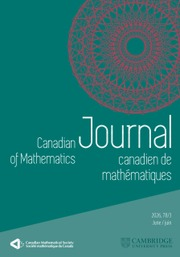Canadian Journal of Mathematics

The Ranks of the Homotopy Groups of a Finite Dimensional Complex

Published online by Cambridge University Press:  20 November 2018

Abstract

HTML view is not available for this content. However, as you have access to this content, a full PDF is available via the ‘Save PDF’ action button.

Let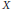$X$ be an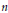$n$ -dimensional, finite, simply connected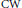$\text{CW}$ complex and set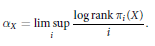$${{\alpha }_{X}}\,=\,\underset{i}{\mathop{\lim \,\sup }}\,\frac{\log \,\text{rank}\,{{\pi }_{i}}\left( X \right)}{i}$$

When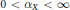$0<{{\alpha }_{X}}<\infty$ , we give upper and lower bounds for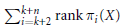$\sum\nolimits_{i=k+2}^{k+n}{\,\text{rank}}\text{ }{{\pi }_{i}}\left( X \right)$ for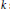$k$ sufficiently large. We also show for any$r$ that$\alpha x$ can be estimated from the integers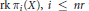$\text{rk }{{\pi }_{i}}\left( X \right),\,i\,\le \,nr$ with an error bound depending explicitly on$r$ .

Type
Research Article
Information
Canadian Journal of Mathematics , 01 February 2013 , pp. 82 - 119

References

 Adams, J. F. and Hilton, P. J., On the chain algebra of a loop space. Comment. Math. Helvetici. 30(1956), 305330. http://dx.doi.org/10.1007/BF02564350 Google Scholar
Anick, D. J., The smallest singularity of a Hilbert series. Math. Scand. 51(1982), no. 1, 3544.Google Scholar
Babenko, I. K., Analytical properties of Poincaré series of a loop space. Mat. Zametki. 27 (1980), no. 5, 751765, 830.Google Scholar
 Félix, Y. and Halperin, S., Rational LS category and its applications. Trans. Amer. Math. Soc. 273(1982), no. 1, 138.Google Scholar
 Félix, Y., Halperin, S., and Thomas, J.-C., The homotopy Lie algebra for finite complexes. Inst. Hautes Études Sci. Publ. Math. 56(1983), 179202.Google Scholar
 Thomas, J.-C., Rational homotopy theory. Graduate Texts in Mathematics, 205, Springer-Verlag, New York, 2001.Google Scholar
 Thomas, J.-C., Exponential growth and an asymptoptic formula for the ranks of homotopy groups of a finite1-connected complex. Ann. of Math. 170(2009), no. 1, 443464. http://dx.doi.org/10.4007/annals.2009.170.443 Google Scholar
 Thomas, J.-C., Lie algebra of polynomial growth. J. London Math. Soc. 43(1991), no. 3, 556566. http://dx.doi.org/10.1112/jlms/s2-43.3.556 Google Scholar
 Friedlander, J. and Halperin, S., An arithmetic characterization of the rational homotopy groups of certain spaces. Invent. Math. 53(1979), no. 2, 117133. http://dx.doi.org/10.1007/BF01390029 Google Scholar
 Gelfand, I. M. and Kirillov, A. A., Sur les corps liés aux algèbres enveloppantes des algèbres de Lie. Inst. Hautes Études Sci. Publ. Math. 31(1966),519 Google Scholar
Gottlieb, D., Evaluation subgroups of homotopy groups. Amer. J. Math. 91(1969), 729756.http://dx.doi.org/10.2307/2373349 Google Scholar
Halperin, S., Torsion gaps in the homotopy of finite complexes. II. Topology 30(1991), no. 3, 471478. http://dx.doi.org/10.1016/0040-9383(91)90026-Z Google Scholar
Krause, G. R. and Lenagan, T. H., Growth of algebras and Gel’fand-Kirillov dimension. Research Notes in Mathematics, 116, Pitman, Boston, MA, 1985.Google Scholar
 Lambrechts, P., Analytic properties of Poincaré series of spaces. Topology 37(1998), no. 6, 13631370. http://dx.doi.org/10.1016/S0040-9383(97)00083-9 Google Scholar
 Lambrechts, P., On the ranks of homotopy groups of two-cones. Topology Appl. 108(2000), no. 3, 303314. http://dx.doi.org/10.1016/S0166-8641(99)00142-X Google Scholar
Milnor, J. and Moore, J. C., On the structure of Hopf algebras. Annals of Math. 81(1965), 211264. http://dx.doi.org/10.2307/1970615 Google Scholar
 van Lint, J. H. and Wilson, R.M., A course in combinatorics. Second ed., Cambridge University Press, Cambridge, 2001.CrossRefGoogle ScholarYou have Access

Send article to Kindle

Note you can select to send to either the @free.kindle.com or @kindle.com variations. ‘@free.kindle.com’ emails are free but can only be sent to your device when it is connected to wi-fi. ‘@kindle.com’ emails can be delivered even when you are not connected to wi-fi, but note that service fees apply.

Find out more about the Kindle Personal Document Service.

The Ranks of the Homotopy Groups of a Finite Dimensional Complex
Available formats
×

Send article to Dropbox

To send this article to your Dropbox account, please select one or more formats and confirm that you agree to abide by our usage policies. If this is the first time you use this feature, you will be asked to authorise Cambridge Core to connect with your <service> account. Find out more about sending content to Dropbox.

The Ranks of the Homotopy Groups of a Finite Dimensional Complex
Available formats
×

To send this article to your Google Drive account, please select one or more formats and confirm that you agree to abide by our usage policies. If this is the first time you use this feature, you will be asked to authorise Cambridge Core to connect with your <service> account. Find out more about sending content to Google Drive.

The Ranks of the Homotopy Groups of a Finite Dimensional Complex
Available formats
×
×